﻿ The Mysterious Constant Alpha (α) in QuantumphysicsPublications are Open
Access in this journal
Article Versions
Export Article
• Normal Style
• MLA Style
• APA Style
• Chicago Style
Research Article
Open Access Peer-reviewed

### The Mysterious Constant Alpha (α) in Quantumphysics

T. G.M. Gerlitz
International Journal of Physics. 2022, 10(1), 59-63. DOI: 10.12691/ijp-10-1-4
Received December 09, 2021; Revised January 14, 2022; Accepted January 23, 2022

### Abstract

The question of how to calculate the numerical value of the fine structure constant from theory was one of the most outstandingly difficult problems in mathematical physics for the greater part of the 20-th century. There were many unsuccessful attempts by researchers including famous ones such as Dirac to find a formula for the fine structure constant. This study is an attempt to demonstrate how to find a mathematical description and to find an idea of the enormous effects this dimensionsless constant has to physics. The formula was found due to the incorporating of the maximal speed a particle can reach. Since this speed is slightly below speed of light but not even relativistic treatment of atoms and other structure must involve corrections that are in its simplest form given by the mysterious factor α called the fine-structure constant. It is shown that the fine-structure constant is a direct consequence of the limited speed of an electrical charged particle in vacuum.

### 1. Introduction

The fine-strucrure constant is a dimensionless physical constant presenting a value for the electromagnetic interaction and plays an important role in classical theories as well as in modern quantum mechanics and QED. It seems to be a kind of key link from the nineteenth century until present including philosophical mysteries (see for an overview. The fine structure constant α, strictly speaking only emerged as a specific entity out of Sommerfeld´s theory for the fine spectroscopy structure of the hydrogen atomic states 1. The birth of the constant occurred in this paper and presents a formula for the Hydrogen spectrum in quantum numbers n, l to expand the energy term in the Bohr´s atom model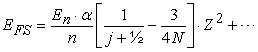(1)

with the physical definition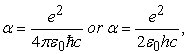(2)

and this is α ≈ 1/137. The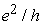is the reciprocal of the von Klitzing´s elementary resistance, which can be determined from the Quantum-Hall effect. The fine-structure constant has several interpretations and some are mentioned here:

1. the square ratio of the elementary charge to the Planck charge

2. the ratio of two energies: (a) the energy needed to overcome the electrostatic repulsion between two electrons a distance d apart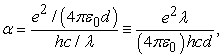(3)

and (b) the energy of a single photon of wavelength λ = 2 π d

3. the ratio of the velocity of the electron in the first circular orbit of the Bohr atom to the propagation speed of light c in vacuum 1.

4. the two ratios of three characteristic lengths: the classical electron radius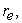the Compton wavelength of the electron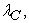and the Bohr radius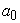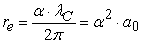(4)

5. in quantum electrodynamics α is directly related to the coupling constant determining the strength of the interaction between electrons and protons. However, QED does not predict its value, and the constant must be gained experimentally. In fact, α is one of the about 20

6. Given two hypothetical point particles each of Planck mass and elementary charge, separated by any distance, then α is the ratio of their electrostatic repulsive force to their gravitational attractice force.

Since the observed value of Alfa (α) is associated with the electron mass, and the electron is lower bound for the energy scale because it – and, of course, the “anti-electron” positron – is the lightet charged object with quantum loops contributing to running, the value 1/137 … is valid at zero energy. Generally, α is seen to be a constant, though, experimental elementary physics shows values differing from the above by more than a magnitude, e.g., on the mass of the Z-boson of 91 GeV revealing a factor of 1/128. Here, it comes to the forth the interaction is screened by electron-positron pairs that exist momentarily out of the vacuum, i. e., vacuum fluctuation. The particles come closer at higher energies and thus there are less electron-positron pairs between them shielding the interaction. The exact value of the constant is defined 2.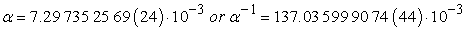(5)

It still stands the question “how can the fine-structure constant value obtained from theory?” Obviously, there was no theory during many decades and probably the reason so few formulae giving values for α following Sommerfeld’s work. There was no idea what forms such a theory would take if it is found; there was also no idea what form any formula that might arise would take or what it would depend on.

A first impressing picture follows from Schroedinger´s theory including the Coulomb potential: the speeds for the Bohr orbits with Z = 1 and Z = 137 are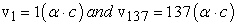(6)

The energies of the first Bohr orbits for various values Z given by n = 1, j = 1/2, and 1 ≤ Z ≤137 is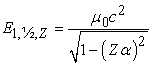(7)

This formula arising from the Bohr theory 3, 4, however, does not take into account relativistic effects (e.g., 5) and especially the lamb shift 6 based on the interaction between the electron and the quantum vacuum 7, 8, 9. An idea is to describe the geometry of particles and fields and construct a mathematical model relating to its charge and spin angular momentum. The spin angular momentum h/4π together with its gyromagnetic factor provides a comparison of the electron charge, therefore Maxwell´s theory and Planck’s constant thus leading to the fine structure constant 2. In the theory of QED a relationship between the dimensionless magnetic moment of the electron and the fine-structure constant is predicted, where the magnetic moment of the electron is referred to as the Landé g-factor.

The most impressing but vivid result from the fine-structure constant is a theoretical chemical element with the fabricated name Feynmanium with the symbol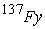and atomic number 137. It was named in honor of Richard Feynman. He noted that a simplistic interpretation of the relativistic Dirac equation 10 runs into problem with electron orbitals at a Z suggesting that neutral atoms cannot exist beyond Feynmanium. Thus, the periodic table of elements must break down at this point. As a already Bohr pointed out his model exhibits difficulty for atoms with atomic number greater than 137 and substantiated this statement by the speed of an electron in a 1s-electron orbital given by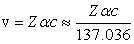(8)

Obviously, this non-relativistic approximation would any element with an atomic number greater than 137 assign a 1s electron a speed faster than light.

Another argument for the above noted limit for the periodic table is given by the relativistic Dirac equation leading to the ground state energy as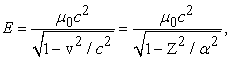(9)

where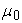is the rest mass of the electron. In the case of Z > 137 the expression under the square root becomes negative and the result must be imaginary. The wave function of the Dirac ground state, then, is oscillatory and there os no gap between positive and negative energy spectra as in the Klein paradox 11.

The aim of the present study is to give an opinion to three phenomena:

1. the fine structure splitting and the correction in the formula by Sommerfeld 1 for the hydrogen atomic state of Bohr 4, which otherwise should allow an electron on its orbit to reach a speed faster than light

2. the Lamb shift 6 justified by an electromagnetic interaction of the electron with the vacuum, and

3. the postulation for a theoretical chemical element of highest possible atomic number, an element 137 in the periodic table 12, also called Feynmanium.

### 2. Theory

The current study is based on recent results found on the discussion of superluminal movements of real particles 13. It is based on the Heisenberg uncertainty and the approximation introduced in the former study of superluminality. Here, In a recent study electrostatic attraction between a subluminal bradyon particle B with its superluminal tachyon co-particle or anti-particle is postulated to posses symmetry with regard to real C-operation, and CPT-operation is required for symmetry conservation for anti-particles. As a consequence of the Lorentz transformation the speed v of the particle observed leads to “deformation” of the electrical field. It has been postulated that under a change from sub- into superluminality the Lorentz transformations change in sign therefore transforming a bradyonic electrical-field vector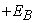into a tachyonic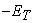entailing the superluminal co-partical T to behave being opposite in electrical charge q to the subluminal particle B in consequence of Coulomb´s law. The transition from subluminal into superluminal state was postulated to occur in no time, the energy borrowed from the quantum vacuum. The present work is to be seen as an exact investigation of the two speeds forming the speed of light, i.e., propagation of light, the lower being v = c – a and the faster of v = c + a . In analogy to the conventional Lorentz factor in Special Relativity it is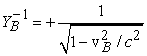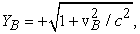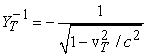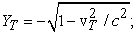for a tachyon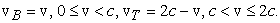(10)

Here, a speed v = c was strictly excluded. Further, the second postulate in Special Relativity exactly spoken is “the speed of light in vacuum is a constant” that means the vacuum propagation (!) of light and nothing else.

The expressions (10) together with the Heisenberg uncertainty were introduced to describe the subject of light-barrier crossing. The relativistic calculation based on the electrostatic interaction between two electrical point charges with non-vanishing rest mass and no spin. The result was a formula to restrict light-barrier crossing of a real particle of point charge q and non-vanishing rest mass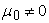to emphasize exactly two speeds leading a particle from a subluminal condition to superluminal: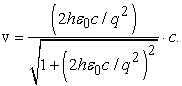(11)

As an example for the elementary charge q = e, negative or positive in sign, the fastest vacuum speed v of a B and the slowest v of a T are then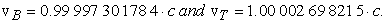(12)

These are the “critical speeds” where jump-over at the light-barrier can occur. As a consequence, a vacuum light-speed c (propagation of light) is therefore never reached by a real particle.

The graphics show this behavior in Figure 1 for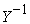and Figure 2 for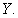It can be seen the traversal between sub- and superluminal states occur at the point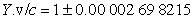marked by an arrow on the the abscissa. Due to the symmetry between the subluminal and superluminal systems only a bradyon B will be considered in the following.

According to the “critical” speed (eg. 11) and the Lorentz´transformation (eg. 10) it is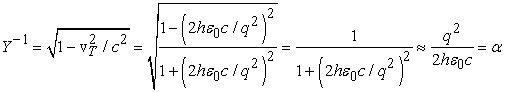(13)

Quod erat demonstandum.

• Figure 1. The factor γ 1 as function of the speed v / c for a particle of the elementary charge e in sub- and superluminal conditions. The arrow on the abscissa marks the speed limits touching the light-barrier
• Figure 2. The factor γ as function of the speed v / c for a particle of the elementary charge e in sub- and superluminal conditions. The arrow on the abscissa marks the speed limits touching the light-barrier

### 3. Results

The general result is that the fine-structure constant is a direct consequence of the speed limit a particle can reach a vaccum, which is not inconsistent with Einstein´s second postulate. Thogh, this postulate often reads “the light-speed in vacuum is a constant” instead of “the speed of light in vacuum is a constant”, the latter means the propagation (!) of light necessary to justify the Lorentz´s transformations.

On the basis of the speed-limit of a particle touching the light-barrier the related value of γ = (1 – vB²/ c²) ≡ α could be calculated. The numerical values are α = 0,007 34 599 01 97 and α –1 = 136,128 68 698 20.

### 4. Discussion

It has to be highlighted the present theory based on a calculation omitting any spin as would be necessary to get a more precise result in the limit-speed a particle can reach. Moreover, in the theory regarding this speed an approximation was used to resolve the equation for the comparison of mechanics with electrodynamics to introduce the Heisenberg´uncertainty. As a consequence, the speed must be slightly faster than used and would lead to an exact expression for α so that an equal sign in eq. (12) becomes true. The value recommended by CODATA 14 is α = 0.007 29 735 25 664 (17).

Principally, this discussion provides an insight into the circumstances that appear in the difficulties and to the discrepancy in atomic models etc. It can be explained how important this factor is, e.g., in atomic physics, just to point out the critics on the incompleteness of the Bohr model 3 so many years ago. That model deals with the problem of the orbit electrons and their, following the theory, a speed faster that light; in their orbits. One of the first modifications suggested by Sommerfeld 1 was the introduction of the fine-structure constant, that results from electrodynamics and relativistic mechanic and leads to a decrease the value in the expression for the atomic energy. Besides those former results the constant plays a central role in QED and, e.g., optical and ESR and NMR spectroscopy (see, e.g., 15, 16) to provide information about chemical coupling and chemical compound in various environments. Due to an inside view α follows a demand for the interaction between a charged (particle) and the quantum vacuum as demonstrated by Lamb and Retherford 6. Since the expression for the speed is exclusively determined by the natural constants e, h. c, h, and εo the constant α itself is independent on any variables as well. An influence to the pure atomic behavior can result in the factor Z given by the number in the atomic table just revealing the statement of a Z = 137 as an upper limit.

### 5. Summary

It has been shown how the fine-structure constant can be proven using the limiting speeds revealed by electrodynamics and relativistic mechanics coupled by the Heisenberg´s uncertainty. Since those speeds are boardering the light-barrier it was taken into account the speed of a real particle, e.g., an electron can never reach speed of light, though, it can come very close to it. The fine-structure constant constitutes this fact so that it is included in other calculation and allows both interpretations and conclusions on physical phenomena. It has been demonstated a value of Z = 137 could be could a sensible guess for the highest atomic number.

### References

  Sommerfeld, A. Zur Quantentheorie der Spektrallinien. Ann. Physik. 51 (1916) 1-94, 125 167. In article View Article  Gabrielse G., Hanneke, D., Kinoshita, T., Nio, M., Odom, B. New Determination of the Fine Structure Constant from the Electron g Value and QED. Phys. Rev. Lett. 97 (2006) 030802. In article View Article  PubMed  Bohr, N. On the constitution of Atoms and Molecules, Part I. Philosophical Magazine 26 (1913) 1-24. In article View Article  Bohr, N. The spectra of helium and hydrogen. Nature 92 (1914) 231-232. In article View Article  Einstein, A. Zum Quantensatz von Sommerfeld und Epstein. Verhandlungen der Deutschen Physikalischen Gesellschaft 19 (1917) 82-92. In article  Lamb, W., Retherford, R. R. C. Fine Structure of the Hydrogen Atom by a Microwave Method. Physical Review. 72 (1947) 241-243. In article View Article  Lamb, W. E., Jr. Anti-photon. Appl. Phys. B. 60. (1995). 77-84. In article View Article  Bethe, H. A., Salpeter, E. E. Quantum Mechanics of One. and Two-Electron Atoms (1957) p. 103. In article View Article  PubMed  Genz, H. Nothingness: the science of empty space. Reading MA, Oxford Perseus (2002) 245 ff. In article  Dirac, P. A. M. The Quantum Theory of the Electron. Proc. Roy. Soc. London, A-778 (1928) 610-624. In article View Article  Klein, O. Die Reflexion von Elektronen an einem Potentialsprung nach der relativistischen Dynamik von Dirac. Zeitschrift fuer Physik. 53 (1929) 3-4. In article View Article  Feynman, R. P. QED: The Strange Theory of Light and Matter. Princeton University Press. (1985) p. 129. In article  Gerlitz, T. G. M. Superluminality and Finite Potential Light-Barrier Crossing. Int. J. Phys. 9 (2021) 234-239. In article View Article  CODATA. Information at the foundation of modern science and technology from the Physical Measurement Laboratory of NIST (2002). In article  Evans, M. W. The role of net angular momentum in pump/probe spectroscopy: Absorption, refringence, scattering, and nuclear resonance. Journal of Molecular Spectroscopy. 146. (1991). 351-368 In article View Article  Carrington A., McLachlan, A. D. Introduction to Magnetic Resonance. John Weatherhill (1967). In articleThis work is licensed under a Creative Commons Attribution 4.0 International License. To view a copy of this license, visit http://creativecommons.org/licenses/by/4.0/